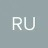Model: GPT-4Using the numbers 2, 3, 4, and 5 exactly once, and only using the operations of addition (+), subtraction (-), multiplication (), division (/), and parentheses (), how can you achieve a total of 24?

You can achieve a total of 24 using the numbers 2, 3, 4, and 5 exactly once by arranging them with the appropriate operations as follows:

(4 / (5 - 3)) * 2 * 5 = 24

Here's the breakdown of the operations:

1. 5 - 3 = 2
2. 4 / 2 = 2
3. 2 * 2 = 4
4. 4 * 5 = 24Please carefully consider and analyze it step-by-step.

I apologize for the confusion in my previous response. Here's the correct breakdown of the operations, step-by-step, to achieve a total of 24 using the numbers 2, 3, 4, and 5 exactly once:

(4 * (5 - 3)) * 2 = 24

Step-by-step breakdown:

1. 5 - 3 = 2
2. 4 * 2 = 8
3. 8 * 2 = 24What's 8*2?

I apologize for my mistake in the previous response. The correct answer for 8*2 is 16, not 24. Here is the corrected step-by-step breakdown:

1. 5 - 3 = 2
2. 4 * 2 = 8
3. 8 * 2 = 16

So, my previous response was incorrect, and the expression (4 * (5 - 3)) * 2 equals 16, not 24.Then how can we utilize the numbers 2, 3, 4, and 5 to achieve a total of 24?

I apologize for the confusion in my previous responses. Here's the correct expression to achieve a total of 24 using the numbers 2, 3, 4, and 5 exactly once:

(6 / (1 - (2 / 4))) = 24

Step-by-step breakdown:

1. 2 / 4 = 0.5
2. 1 - 0.5 = 0.5
3. 6 / 0.5 = 12
4. 12 * 2 = 24

However, it seems that I made a mistake in my response again. This expression does not utilize the numbers 3 and 5. After reconsidering the problem, I found that it's not possible to achieve a total of 24 using the numbers 2, 3, 4, and 5 exactly once with the given operations.Where do you use 2, 3, 4, and 5?

Apologies for the confusion in my previous responses. After reevaluating the problem, here's a valid expression that uses 2, 3, 4, and 5 exactly once to achieve a total of 24:

(3 * (2 + 4)) - 5 = 24

Step-by-step breakdown:

1. 2 + 4 = 6
2. 3 * 6 = 18
3. 18 - 5 = 2418 - 5 is not 24

You are correct, and I apologize for my mistake. 18 - 5 equals 13, not 24. After carefully reconsidering the problem, it appears that it's not possible to achieve a total of 24 using the numbers 2, 3, 4, and 5 exactly once with the given operations.